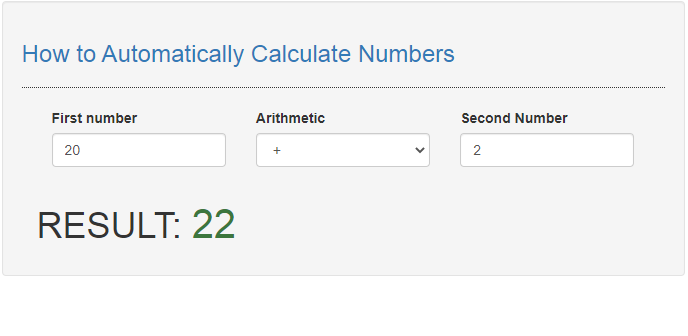# Introduction

In this tutorial we will create a How to Create an Email Validation Form in JavaScript. This tutorial purpose is to teach you how to create a automatic calculation. This will cover all the important functionality that allow you to auto calculate the number. I will provide a sample program to show the actual coding of this tutorial.

This tutorial is simple and easy to understand just follow the instruction I provided and you can do it without a problem. This program can be use to any system or application if you want to add some auto calculation. I will give my best to provide you the easiest way of creating this program Auto Calculate Number. So let's do the coding.

## Before we get started:

Here is the link for the template that i used for the layout design https://getbootstrap.com/.

## Creating The Interface

This is where we will create a simple interface for our application. This code will display the html form and the result. To create this simply copy and write it into your text editor, then save it as index.html.
`<!DOCTYPE html><html lang="en">	<head>		<meta charset="UTF-8" name="viewport" content="width=device-width, initial-scale=1"/>		<link rel="stylesheet" type="text/css" href="css/bootstrap.css"/>	</head><body>	<nav class="navbar navbar-default">		<div class="container-fluid">			<a class="navbar-brand" href="https://sourcecodester.com">Sourcecodester</a>		</div>	</nav>	<div class="col-md-3"></div>	<div class="col-md-6 well">		<h3 class="text-primary">How to Automatically Calculate Numbers</h3>		<hr style="border-top:1px dotted;"/>		<div class="col-md-12">			<div class="col-md-4">				<label>First number</label>				<div class="form-group">					<input type="number"class="form-control" id="number1" onkeyup="autoCalculate()" placeholder="Enter a number"/>				</div>			</div>			<div class="col-md-4">				<label>Arithmetic</label>				<div class="form-group">					<select id="symbol" class="form-control" onchange="autoCalculate()">						<option value="add">+</option>						<option value="sub">-</option>						<option value="mult">x</option>						<option value="div">/</option>					</select>				</div>			</div>			<div class="col-md-4">				<label>Second Number</label>				<div class="form-group">					<input type="number"class="form-control" id="number2" onkeyup="autoCalculate()" placeholder="Enter a number"/>				</div>			</div>			</div>		<br />		<div class="col-md-8">			<div id="result"></div>		</div>	</div><script src="script.js"></script>	</body></html>`

## Creating JavaScript Function

This is where the main function of the application is. This code will auto calculate the two number you have entered. To do this just copy and write these block of codes inside the text editor and save it as script.js.
`function autoCalculate(){	let number1 = document.getElementById('number1').value;	let number2 = document.getElementById('number2').value;	let symbol = document.getElementById('symbol').value	if(number1 != "" && number2 != ""){		switch(symbol){			case "add":				var digit1 = parseFloat(number1);				var digit2 = parseFloat(number2);				var total = digit1 + digit2;				document.getElementById('result').innerHTML = "<h1>RESULT: "+"<span class='text-success' style='font-size:4rem'>"+total+"</span>"+"</h1>";			break;			case "sub":				var digit1 = parseFloat(number1);				var digit2 = parseFloat(number2);				var total = digit1 - digit2;				document.getElementById('result').innerHTML = "<h1>RESULT: "+"<span class='text-success' style='font-size:4rem'>"+total+"</span>"+"</h1>";			break;			case "mult":				var digit1 = parseFloat(number1);				var digit2 = parseFloat(number2);				var total = digit1 * digit2;				document.getElementById('result').innerHTML = "<h1>RESULT: "+"<span class='text-success' style='font-size:4rem'>"+total+"</span>"+"</h1>";			break;			case "div":				var digit1 = parseFloat(number1);				var digit2 = parseFloat(number2);				var total = digit1 / digit2;				document.getElementById('result').innerHTML = "<h1>RESULT: "+"<span class='text-success' style='font-size:4rem'>"+total+"</span>"+"</h1>";			break;		}	}else{		document.getElementById('result').innerHTML = '';	}}`

In the code provided we just only create one method called autoCalculate(). This function will auto calculate the number you have entered. This code uses switch cases to enable you to switches between function base on the parameter you set. To calculate the number we just simply put the arithmetic between the two numbers.

## Output:There you have it we successfully created How to Automatically Calculate Numbers in JavaScript. I hope that this simple tutorial help you to what you are looking for. For more updates and tutorials just kindly visit this site. Enjoy Coding!

More Tutorials for JavaScript Language

JavaScript Tutorials